• Blog

# Narrowing the Search: Which Hyperparameters Really Matter?

Tech Blog Aimee CoelhoHyperparameter optimization feels like figuring out a map on a budget (Credit: Pexels)

## How Can You Identify Which Hyperparameters Are Important?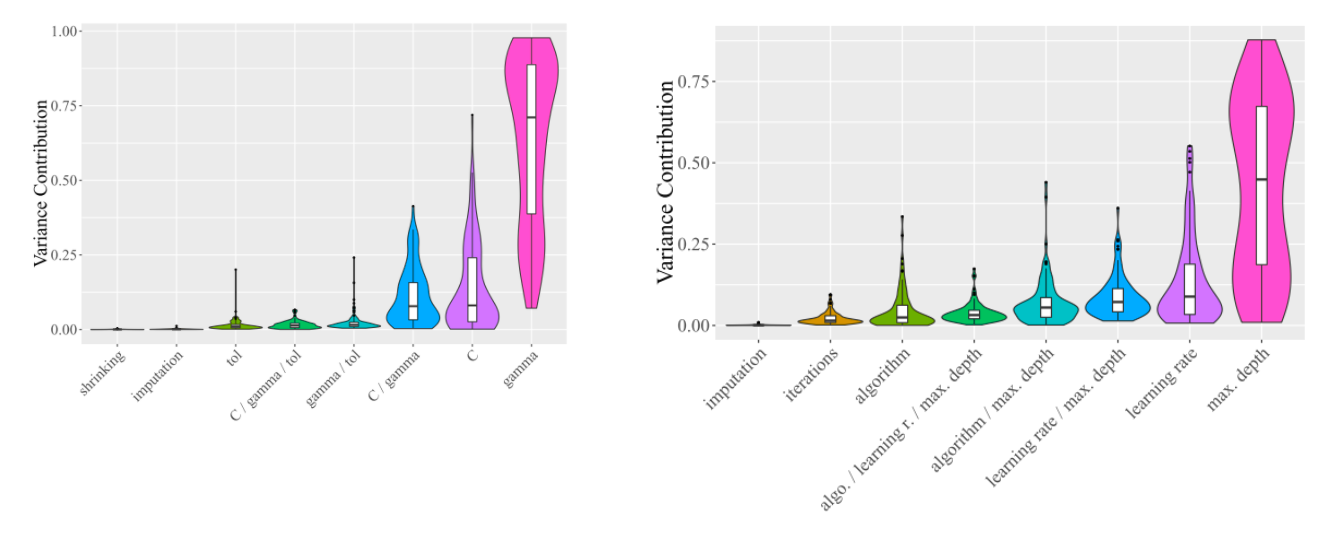Figure 1: From the original paper, the marginal contributions over all datasets for SVM (RBF Kernel) (left) showing that hyperparameter gamma was the most important and AdaBoost (right) showing that max depth was the most important hyperparameter.

## Hyperparameter Importance Density Distributions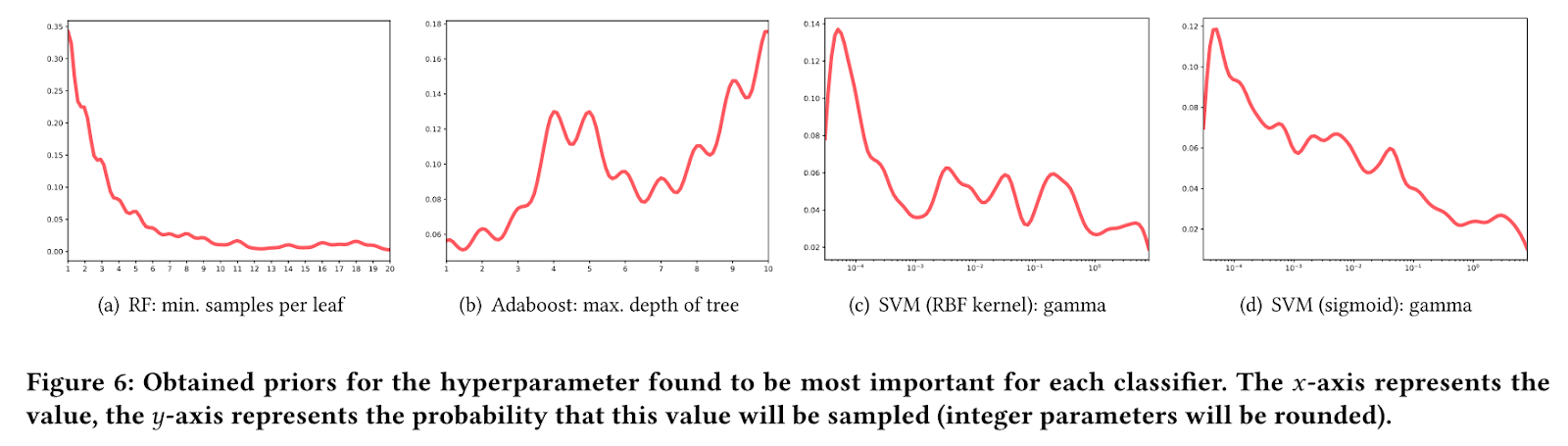Figure 2: Examples of density distributions of the top ten evaluations from all datasets for some of the hyperparameters of the algorithms studied in the paper, Random Forest, Adaboost, SVM (RBF kernel) and SVM (sigmoid).

## More Random Forest Hyperparameters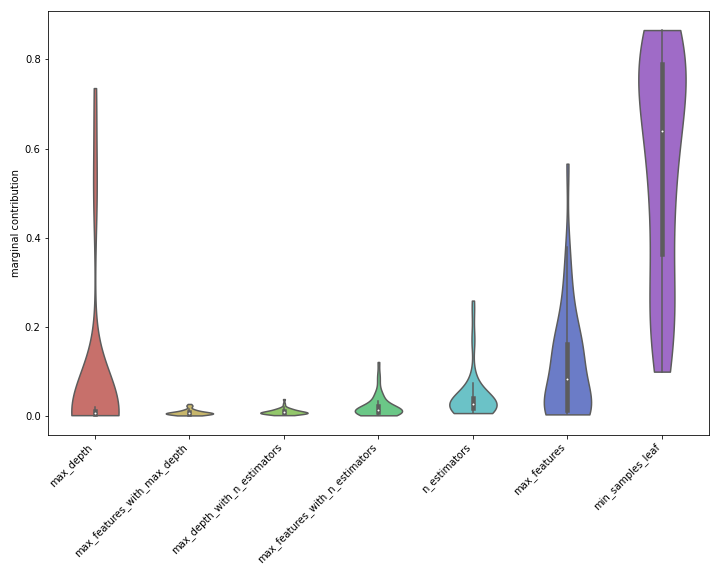Figure 3: The violin plot of importances for this set of hyperparameters for random forest, showing min samples leaf to be by far the most important hyperparameter generally.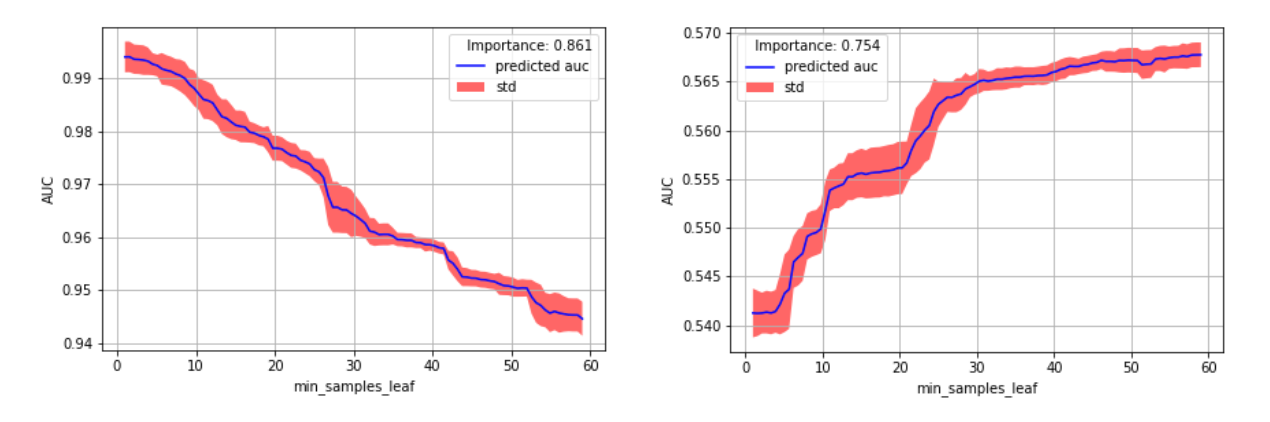Figure 4: The marginal performance for min samples leaf for random forest on the mice protein dataset and on the analcatdata DMFT dataset where higher values of min samples leaf are better.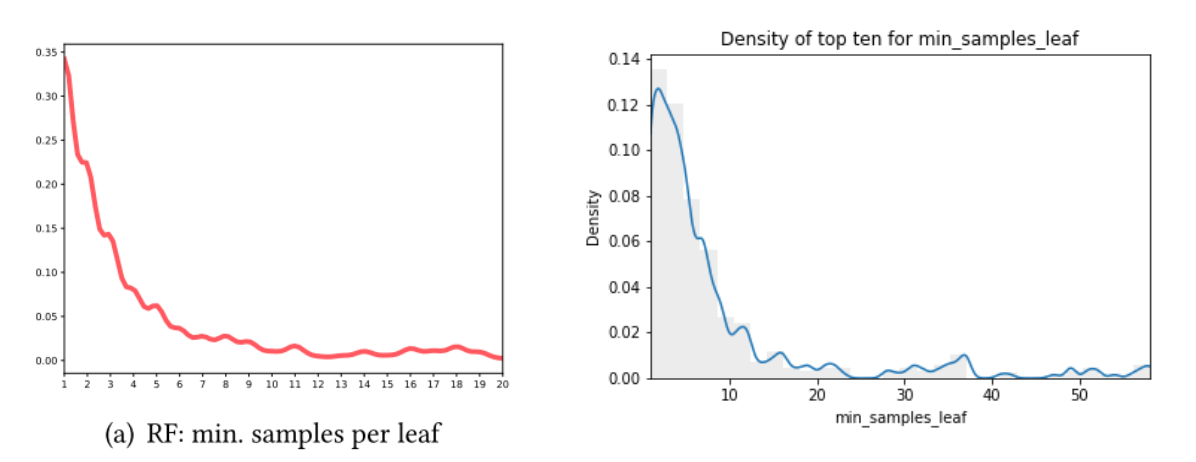Figure 5: One-dimensional density plots of the 10 best values of min samples leaf across all datasets, from the original paper (left) and for our new experiments on OpenML-CC18 data with different hyperparameters (right).

## XGBoost Experiments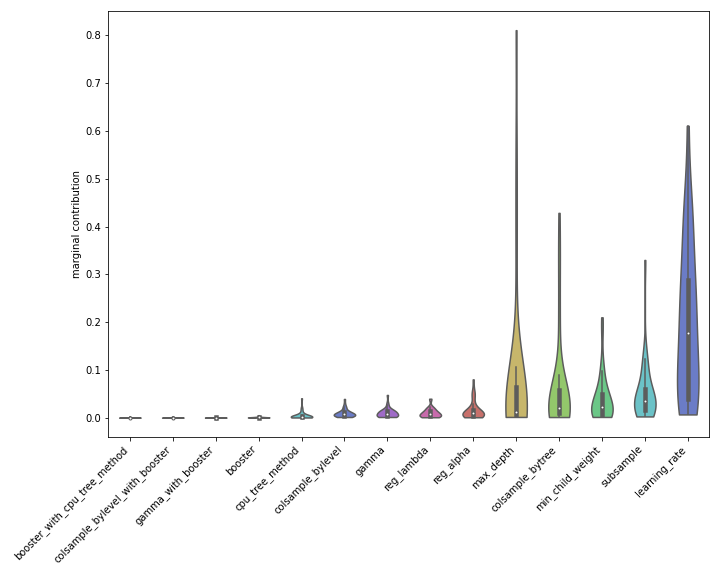Figure 6: A violin plot showing the most important hyperparameters and interactions for XGBoost over all datasets with learning rate generally the most important.

#### Important Hyperparameters for XGBoost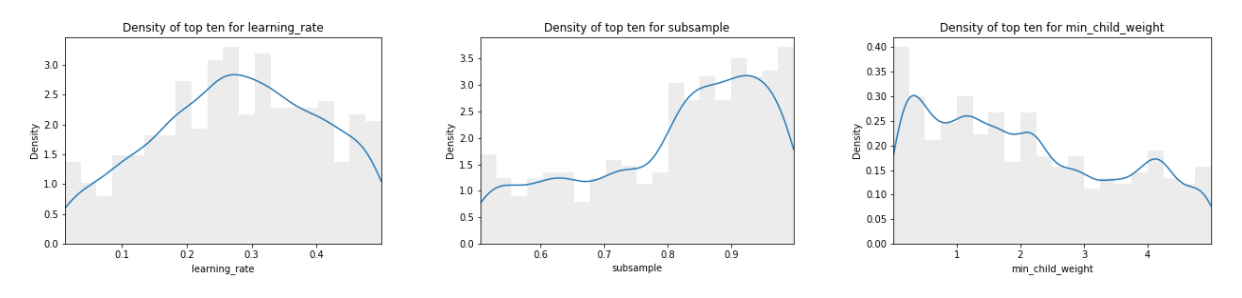Figure 7: The density distributions of the ten best values over all datasets for the hyperparameters learning rate, subsample and min child weight for XGBoost.

## What Are Our Takeaways From This Experiment?

#### How to Set the Number of Estimators?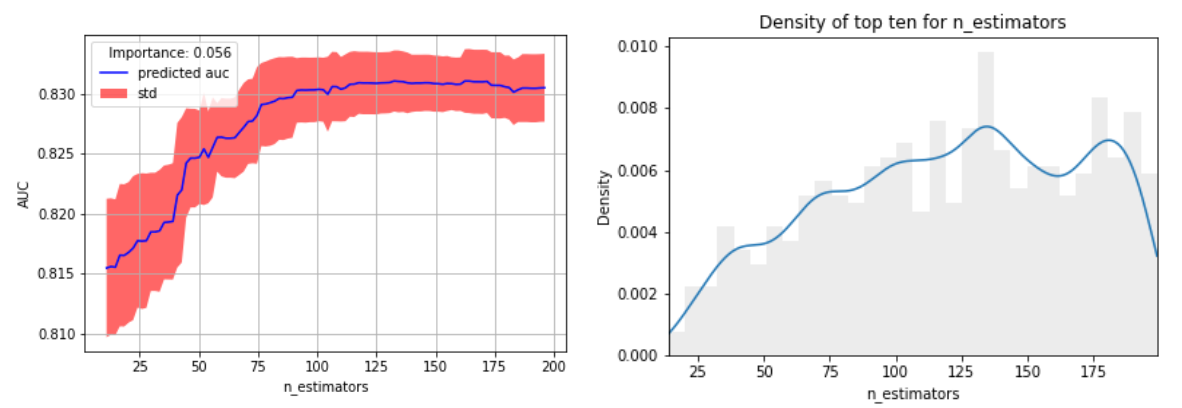Figure 8: An example marginal for the number of estimators parameter for random forest on the madelon dataset, and the density distribution of the best values of n estimators over all datasets.